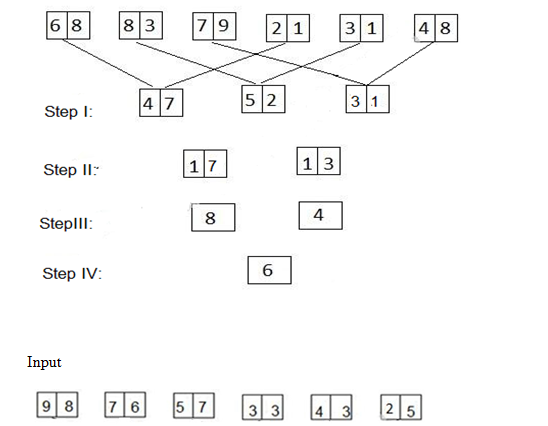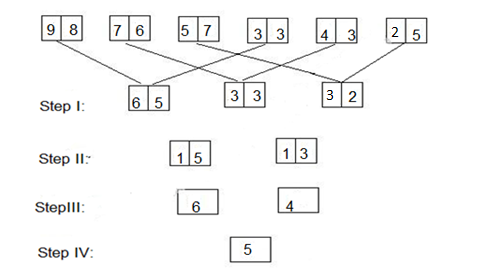# Crack SBI Clerk / IDBI Executive 2018 – Logical Reasoning (Coding Decoding and Input Output) Day-70

Dear Readers, Reasoning Section play a vital role in Banking and all other competitive exams. This year 2018 was started with three wonderful opportunities; Most Awaited SBI Clerk 2018. To enrich your preparation here we have providing new series of Practice Questions on Reasoning Ability – Coding Decoding and Input Output. Candidates those who are going to appear in Bank Exams 2018 can practice these questions daily and make your preparation effective.

[WpProQuiz 2116]

Click “Start Quiz” to attend these Questions and view Explanation

1) In a certain code, a number 18462 is written as BETKO and 7935 is written as RAHU. How is 43857 written in that code?

a) THOEB

b) THROB

c) THKOB

d) THEUR

e) None of these

2) In the following sequence or instructions, 6 stands for Run, 7 stands for Stop, 8 stands for Go, 9 stands for Sit and 0 stands for Wait. If the sequence is continued, which instruction will come next?

9 9 0 9 0 8 9 0 8 6 9 0 8 6 7 9 0 9 0 8 9 0 8

a) Wait

b) Sit

c) Stop

d) Run

e) None of these

3) If the first and second letters in the word CONCESSION were interchanged, also the third and the fourth letters, the fifth and the sixth letters and so on, which of the following would be the seventh letter from the right ?

a) R

b) O

c) S

d) N

e) None of these

4) In a certain code ‘na pa ka so’ means ‘Rose is red colour’, ‘ri so la pa’ means ‘Jasmine likes rose colour’ and ‘ti me ka bo’ means ‘Blood also in red’. Which of the following is the code for ‘is’in that language?

a) na

b) ka

c) bo

d) so

e) None of these

5) If ‘P’ denotes ‘–’; ‘Q’ denotes ‘ ÷ ‘, ‘R’ denotes ‘×’ and ‘W’ denotes ‘+’ then  48 Q 12 R 10 P 8 W 4 = ?

a) 56

b) 40

c) 52

d) 44

e) None of these

Directions (6-10): A string of numbers is given as input. The further steps given are obtained by applying certain logic. Numbers of step II have been obtained by using at least 1 digit of each number in step I. Each step is a resultant of previous step only.6) Which number is smallest in step 1?

a) 53

b) 33

c) 32

d) 65

e) None of these

7) What is the second smallest number obtained in 2nd step of given input?

a) 85

b) 22

c) 15

d) 13

e) None of these

8) Find the difference between sum of numbers obtained in 1st step and sum of numbers obtained in 2nd step.

a) 127

b) 67

c) 102

d) 72

e) 76

9) What is the difference between the second largest number and the smallest number obtained in 1st step?

a) 1

b) 8

c) 5

d) 9

e) 2

10) What is the average of the numbers obtained in last 2 steps?

a) 4

b) 5

c) 9

d) 7

e) None of these

1  8  4  6  2         7   9   3   5

B E T  K O        R   A  H  U

4   3  8   5  7

T  H  E  U  R

9, 90, 908, 9086, 90867, 90, 908, 9086

The next coded digit will be 6. Hence, the instruction

Run will come next.

The new letter sequence is OCCNSEISNO

The seventh letter from the right is N.

O C C  N S E  I  S N O

1  2  3  4  5 6  7  8  9 10

na pa ka so = Rose is red colour

ri so la pa = Jasmine likes rose colour

ti me ka bo = Blood also in red

Thus “is” coded as “na”.

5. Answer e) None of these

48 Q 12 R 10 P 8 W 4 = ?

? = 48 ÷ 12 × 10 – 8 + 4

? = 4 × 10 – 8 + 4

? = 40 – 8 + 4 = 36

Direction (6-10)For given input:

Step I: 65…..…33……..32 — Subtraction

Step II: 15……13(6*3 – 3 = 15) &(5*3-2 =13)

Step III: 6…….4 –Addition (1+5=6) & (1+3= 4)

Step IV: 5— Average ((6+4)/2 =5)

Required difference = (65+33+32) – (15+13) = 102

Required difference = 33 –32 = 1